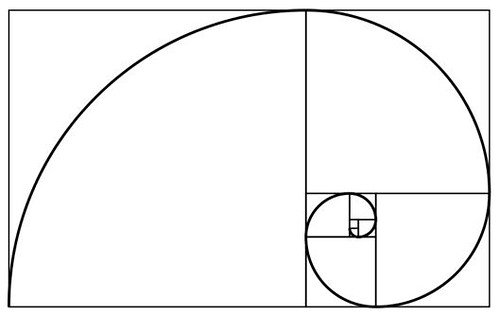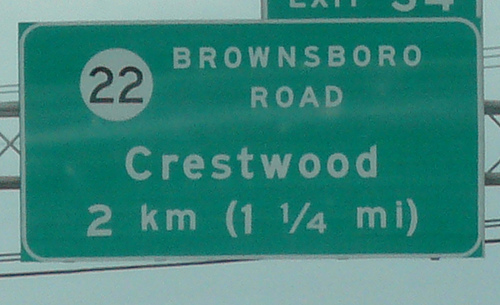# (Roughly) Daily

## Spiraling into control…The Fibonacci spiral (source)

As we remark that math really is beautiful, we might send elegantly parsimonious birthday greetings to one of Fibonacci’s spiritual descendants, a father of Pure Mathematics, Leonhard Euler; he was born on this date in 1707.  While crafting “the most remarkable formula in mathematics,” Euler made foundational contributions to number theory, graph theory, mathematical logic, and applied math; he originated many commonly-used figures of mathematical notation, and invented the concept of the “mathematical function.”  And he was no slouch in physics either, making renowned contributions in work in mechanics, fluid dynamics, optics, and astronomy.source

Written by (Roughly) Daily

April 15, 2012 at 1:01 am

Posted in Uncategorized

## Knowing the Distance: More Fun With Numbers…

The Fibonacci sequence describes the golden ratio (or golden spiral), an ideal form found in the more beautiful corners of nature, and much beloved by designers everywhere.

The Fibonacci numbers are the sum of the previous two numbers in the sequence, starting with 0 and 1:  0, 1, 1, 2, 3, 5, 8, 13, 21, 34, 55, 89, 144…A Fibonacci spiral created by drawing circular arcs connecting the opposite corners of squares in a Fibonacci tiling; this one uses squares of sizes 1, 1, 2, 3, 5, 8, 13, 21, and 34. (source)

It turns out that the Fibonacci sequence also neatly matches the relationship between kilometers and miles. Three miles is five kilometers, five miles is eight kilometers, eight miles is 13 kilometers.  It’s not perfect: eight miles is actually 12.875 kilometers– but it’s close enough in a pinch.

If one needs to convert a number that’s not in the Fibonacci sequence, one can simply break out the Fibonacci numbers, convert, and add the answers.  For instance, 100 can be broken down into 89 + 8 + 3, all Fibonacci numbers. The next numbers are 144, 13, and 5, which add up to 162. 100 miles is actually equal to 160.934 kilometers.  But again, close enough.photo: Matt Hampel

[TotH to MNN]

Special bonus arithmetic amusement:  the quadratic equation, explained (as though) by Dr. Seuss.

As we marvel at math, we might wish a Happy Birthday to a master of “numbers” of a different sort; author and prankster Ken Kesey was born on this date in 1935.  While at Stanford in 1959 (studying writing with Wallace Stegner), Kesey was a paid volunteer in CIA-funded LSD trials (Project MKULTRA), an experience that informed his novel, One Flew Over the Cuckoo’s Nest, and that inspired him to form the “Merry Pranksters” and embark on the cross-country school bus trip memorialized in Tom Wolfe’s “Electric Kool-Aid Acid Test.”

“Leave no turn unstoned.”source

Written by (Roughly) Daily

September 17, 2011 at 1:01 am